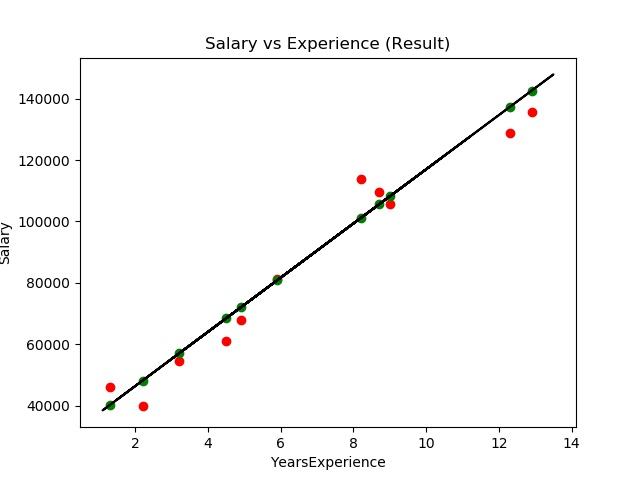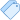June 19, 2020 Deepak Raj

## Introduction

The term regression was first applied to statistics by the polymath Francis Galton. Galton is a major figure in the development of statistics and genetics.

Linear Regression is one of the simplest machine learning algorithms that map the relationship between two variable by fitting a linear equation to observed data. One variable is considered to be an explanatory variable, and the other is considered to be a dependent variable.

## Simple Linear Regression

In simple linear regression when we have a single input. and we have to obtain a line that best fits the data. The best fit line is the one for which total prediction error (all data points) are as small as possible. Error is the distance between the point to the regression line.

E.g: Relationship between hours of study and marks obtained. The goal is to find the relationship between the hours of study and marks obtained by the student. for that, we have to find a linear equation between these two variables.

$$y(predict) = b_0 + b_1 *x$$

Error is the difference between predicted and actual value. to reduce the error and find the best fit line we have to find value for bo and b1. $$Error = (Y_p - Y_a) ^ 2$$ for finding a best fit line value of bo and b1 must be that minimize the error.error is the difference between predicted and actual output.## Assumptions of simple linear regression

Simple linear regression is a parametric test, meaning that it makes certain assumptions about the data. These assumptions are:

• Homogeneity of variance (homoscedasticity): the size of the error in our prediction doesn’t change significantly across the values of the independent variable.
• Independence of observations: the observations in the dataset were collected using statistically valid sampling methods and there are no hidden relationships among observations.
• Normality: The data follows a normal distribution.
• The relationship between the independent and dependent variable is linear: the line of best fit through the data points is a straight line (rather than a curve or some sort of grouping factor). If your data do not meet the assumptions of homoscedasticity or normality, you may be able to use a nonparametric test instead, such as the Spearman rank test.

## Linear Regression example

Let us now apply Machine Learning to train a dataset to predict the Salary from Years of Experience.

## Importing Libraries and Datasets

Three python libraries will be used in the code.

• pandas
• matplotlib
• sklearn

## Split the Data

In this step, Split the dataset into the Training set, on which the Linear Regression model will be trained and the Test set, on which the trained model will be applied to visualize the results. In this the test_size=0.3 denotes that 30% of the data will be kept as the Test set and the remaining 70% will be used for training as the Training set.

## Fit and Predict

Import LinearRegression from linear_model and assigned it to the variable lr. lr.fit() used to learn from data and lr.predict() used to predict basis on learn data.

## Evaluate and Visualize

Create a pandas dataframe of predict and actual values and visualize the dataset.## Conclusion

Thus in this story, we have successfully been able to build a Simple Linear Regression model that predicts the ‘Salary’ of an employee based on their ‘Years of Experience’ and visualize the results.tags: machine learning, data science, python